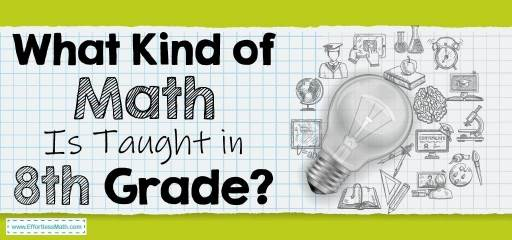# What Kind of Math Is Taught in 8th Grade?Math is one of the most important subjects in school. Students use math to learn how to problem-solve, analyze data, and much more! It’s also a subject that can be challenging for many students. But don’t worry— there are plenty of helpful resources out there that may help you better prepare for your classes or just brush up on some skills!

The kind of math that is taught in 8th grade varies depending on the country. In many countries, students are expected to learn advanced algebra at this level, which includes solving word problems.

They also have to know how to use an advanced calculator and find out how many decimal places they need for their answer.

The Absolute Best Book to Ace the 8th Grade Common Core Math Test

## What Math Should an 8th Grader Know?

While the curriculum will vary from region to region and in some cases institution to institution, here’s a list of topics and skills that may be covered:

• Multiplication tables
• Fractions—decimal equivalents, ordering fractions from least to greatest, converting between fractional and decimal forms. This also includes learning how to find equivalent fractions such as ¾ = 0/75 or 16/25.
• Geometry concepts like angles, triangles, quadrilaterals (including knowing what makes them different), perimeter calculations for geometric shapes with easy side lengths are given. They should know about circles too including circumference and area! The students should learn prime factorization at this level as well as do some other more complicated factoring.
• Advanced algebra—word problems, factoring polynomials up to the third degree (highest), solving quadratic equations and inequalities by completing the square, graphing lines including slope/intercepts/equations of a line, understanding how changing one variable affects other variables in an equation. This also includes linear systems including the distance formula & midpoint formula. They should know how to solve multiple-step word problems as well! Don’t worry though because there are plenty of practice questions you can use if you’re struggling with these skills yourself!

## Math Objectives for 8th Grade

In the 8th grade, students have already reached an age where they get to do analytical problems. So the main objective in 8th grade is to sharpen the minds of students even further. The other objectives include;

• Make students comfortable with all topics covered in the school.
• Develop good problem-solving abilities and critical thinking skills.
• Help students understand concepts & build a strong foundation for future learning.
• To know the difference between rational and irrational numbers.
• Know and perform the different transformations of any given figure on a coordinate plane.
• Solve different mathematical problems using linear inequalities.
• learn to use sampling techniques.

These are just some of the main objectives that teachers have when teaching math to their students so it is important to know what your kid’s teacher expects from them!

The Best Books to Ace the 8th Grade Common Core Math Test

### What people say about "What Kind of Math Is Taught in 8th Grade? - Effortless Math: We Help Students Learn to LOVE Mathematics"?

No one replied yet.

X
24% OFF

Limited time only!

Save Over 24%

SAVE \$5

It was \$20.99 now it is \$15.99